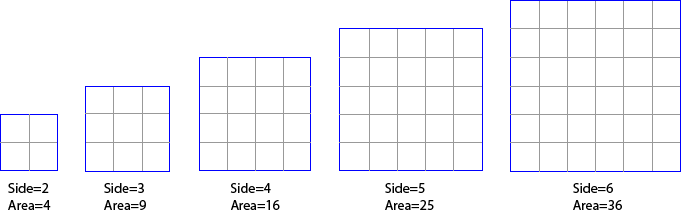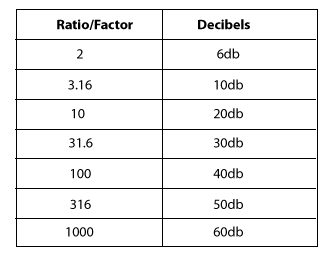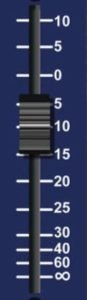HomeUnderstanding AudioUnderstanding Decibels (part 1)

# Understanding Decibels (part 1)

Decibels are widely used in audio, and often misunderstood. These articles give a practical understanding on using decibels in audio work.  But first, some basic questions and answers about decibels.

### What is a decibel?

A decibel is a tenth of a Bel, a unit of level, named after Alexander Graham Bell. A Bel is a very large unit, so the prefix deci (one tenth) is used. A decibel uses a logarithmic scale, not a linear scale like volts or watts – see below.

There is no absolute level called a decibel. A decibel expresses a ratio. It is relative to something. Unfortunately, what it is relative to is often not mentioned when stating a decibel reading. For example, the line out of mixer might be at -10dB, this usually means it is 10dB below 0dB.

### Why Decibels?

So why use a decibel? You might have noticed that the volume control on most HiFi amps is marked in decibels, as is the markings on mixer level controls. This is because our range of hearing is so vast, that to use a linear scale we would be using figures from 0 – 1,000,000!

#### Decibels are non linear

A secret to understanding decibels is to note that decibels are non linear. Another example of a non-linear relationship is between the side of a square and the area of a square.In this example, you can see that increasing the side measurement does not have an equivalent increase in area, but a larger increase.  Also, a doubling in the side length does not double the area – it is a lot more than the double! This is an example of a non-linear relationship – in this case, a small increase in the side relates to a different increase in the area. Decibels are similar. A small change in decibels relates to a different change in the ratio of the two levels being compared.

### Decibels Express a Ratio

When we are talking about audio levels, we are looking at voltages, or sound wave amplitudes. (Note: power measurements (like the power differences in an amplifier) use a similar but different formula). But without getting into formulas etc, we need to accept the following summary of linear ratios of voltages and decibels. (I’m not showing the formulas or calculations because I reckon most people skip over them anyhow, and if you like formulas any search engine will give you as many as you like)There are some interesting points highlighted by this table:

• Double the voltage (x2) changes the output by 6dB (see examples below)
• The easy ones to remember are x10 = 20dB, x100 = 40dB and x1000 = 60dB

For those who want to see other ratios and decibel values, you can use this simple calculator. Otherwise, just skip the calculator and read the examples below to help understand how decibels are used.

Example One: you increase the volume on a mixer or amplifier by 6dB: this is actually doubling the voltage that will appear at the output (because 6dB is a factor of 2 – see table). So, we could say the level is now +6dB. That is, relative to what the level was, it is now 6dB higher. Remember decibels are always relative to something, in this case, to what it was before we doubled (added 6dB to) the level.

Example Two: same scenario, but this time we lower the volume by 6dB. This means we are halving the voltage (because 6dB is a factor of 2). So, we could say the output is now -6dB (below what it was before).

These examples also show that it is possible to have positive and negative decibels. If the voltage is +6dB, it is twice the size as it was before. If is it -6dB, then it is half the size it was before.

I know it can be hard, but stay with me here, a few more examples should help.

Let’s say we have a microphone that is giving out 1 millivolt (that is, a thousandth of a volt) when we speak into it. We plug this microphone into a pre-amp (microphone input on a camera or mixer) that has a gain of 60dB. From the table above, we see that 60dB is equal to a linear ratio of 1000. Therefore, a pre-amp with a gain of 60dB is going to amplify our 1mV mic level signal by a factor of 1000, giving us a level of 1,000 millivolts or 1 volt. Behold, we now have a signal which is at line level (see article on Audio Levels).

Consequently, we could say that the level of the microphone signal is -60dB compared to the amplified line level. That is, the input is 1000th of what the output of the pre-amp is.

In practice, most microphones will produce about 10 millivolts (when spoken directly in to), so the signal only needs to be amplified by a factor of 100 to make it up to 1 volt (0.01 x 100 = 1.0). The table above tells us that a factor of 100 is 40dB.

Most mic pre-amps should amplify the signal by at least 40 dB (x100) and some will amplify up to 60dB (x1000). Most mixers have a gain control at the top of each channel. This will allow you to vary the gain of the preamp, to better match the input level.

Some video cameras (semi-professional or professional) will have a switch which allows you to switch the gain between 40dB, 50dB or 60dB gain for the preamp. The other way of stating this is to say the input is selected for -40dB, -50dB or -60dB levels. Just remember it is all relative, and the greater the number, the greater the amplification.

Have a look at the manual for your HiFi, mixer or video camera, and find out what level your mic input it expecting. If you are putting more in, it will probably cause distortion.

If for instance you are trying to connect a mixer (PA board) tape out, into your mic input, what do you need to consider?  Well, first we need to know what the output level is likely to be. Let’s say that the specifications say the tape out (line out) is  -10dBV or 316 millivolts and your mic input is looking for -50dBV (or 3.16 millivolts).  This is where decibels makes it easy. We have a level of -10dB, and need to get it to -50dB, easy, use a 40dB attenuator to reduce the level. That is easier than calculating the ratio between 316mV and 3.16mV, (which when you calculate it happens to come to a linear ratio of 100 or a logarithmic ratio of 40dB).

Again, a 40 dB attenuator is indicating a ratio, in this case the ratio between the signal in and the signal out is 40dB, or a factor of 100. That is, the output is one hundredth of the input. If you want to look at other voltage gain or attenuation ratios and decibels, have a play with my Decibel Calculator for Audio

Decibels do make calculations easy, but it can be difficult to comprehend.

In the next article we look at how to read the specifications about decibels, before looking at some real world applications.